﻿ 基于图像插值误差扩展的可逆医学图像水印 Reversible Medical Image Watermarking Based on Image Interpolation Error Expansion

Computer Science and Application
Vol.08 No.04(2018), Article ID:24527,7 pages
10.12677/CSA.2018.84053

Reversible Medical Image Watermarking Based on Image Interpolation Error Expansion

Yue Chang, Xuan Wang

Shaanxi Normal University, Xi’an Shaanxi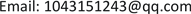Received: Apr. 3rd, 2018; accepted: Apr. 19th, 2018; published: Apr. 26th, 2018ABSTRACT

Embedding watermark information into audio, image or video digital signals can protect the copyright information of products. After the watermark information is extracted, the original image can be restored without any distortion in the reversible watermark image. In this paper, we use the additive interpolation error extension method to embed watermark data into the smooth region of the image. Unlike previous watermarking methods, the additive interpolation error expansion embeds bits “1” or “0” by adding or expanding the interpolation error additively. Since only slight modifications have been made to the pixels of the smooth area, the high quality of the image is preserved. The experiment results show that the proposed scheme can provide greater payload capacity and higher image fidelity, compared with other most advanced schemes.

Keywords:Medical Image, Interpolation Image, Additive Interpolation Error Expansion, Reversible Watermark1. 引言

2. 加法插值误差扩展

$e=X-{X}^{\prime }$ (1)

${e}^{\prime }=\left\{\begin{array}{ll}e+sign\left(e\right)×b,\hfill & e=LM/RM\hfill \\ e+sign\left(e\right)×1,\hfill & e\in \left(LN,LM\right)\cup \left(RM,RN\right)\hfill \\ e,\hfill & \text{otherwise}\hfill \end{array}$ (2)

$sign\left(e\right)=\left\{\begin{array}{ll}1,\hfill & e\in LE\hfill \\ -1,\hfill & e\in RE\hfill \end{array}$ (3)

$\left\{\begin{array}{l}LN=\mathrm{arg}\underset{e\in LE}{\mathrm{min}}hist\left(e\right)\\ RN=\mathrm{arg}\underset{e\in RE}{\mathrm{min}}hist\left(e\right)\end{array}$ (4)

${X}^{″}={X}^{\prime }+{e}^{\prime }$ (5)

${e}^{\prime }={X}^{″}-{X}^{\prime }$ (6)

$b=\left\{\begin{array}{ll}0,\hfill & {e}^{\prime }=LM/RM\hfill \\ 1,\hfill & {e}^{\prime }=LM-1/RM+1\hfill \end{array}$ (7)

$e=\left\{\begin{array}{ll}{e}^{\prime }-sign\left({e}^{\prime }\right)×b\hfill & {e}^{\prime }\in \left[LM-1,LM\right]\cup \left[RM,RM+1\right]\hfill \\ {e}^{\prime }-sign\left({e}^{\prime }\right)×1\hfill & {e}^{\prime }\in \left[LN,LN-1\right]\cup \left[RM+1,RN\right]\hfill \\ e\text{'}\hfill & \text{otherwise}\hfill \end{array}$ (8)

$X={X}^{\prime }+e$ (9)

3. 插值算法

${X}_{h}\left(2i,2j\right)$ 在由四个低分辨率像素围绕的中心位置处被内插。其次，其他缺失的像素 ${X}_{h}\left(2i-1,2j\right)$${X}_{h}\left(2i,2j-1\right)$ 在已经恢复的像素的帮助下进行插值预测。如图1(b)所示，我们可以沿着45°和135°两个方向对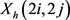进行插值预测。预测过程如下：

$\left\{\begin{array}{l}{{X}^{\prime }}_{45}=\left({X}_{l}\left(i,j+1\right)+{X}_{l}\left(i+1,j\right)\right)/2\hfill \\ {{X}^{\prime }}_{135}=\left({X}_{l}\left(i,j\right)+{X}_{l}\left(i+1,j+1\right)\right)/2\hfill \end{array}$ (10)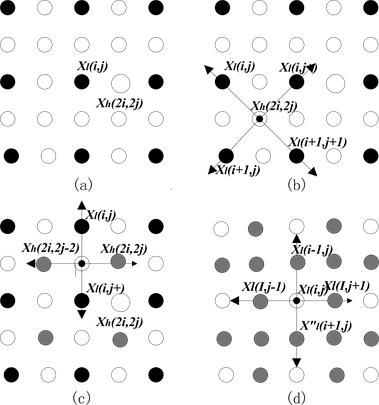Figure 1. (a) Formation of a low-resolution image from the high-resolution image; (b), (c) interpolation of residual samples of high-resolution; (d) the interpolation of the original pixels

$\left\{\begin{array}{l}{e}_{45}\left(2i,2j\right)={{X}^{\prime }}_{45}\left(2i,2j\right)-{X}_{h}\left(2i,2j\right)\hfill \\ {e}_{135}\left(2i,2j\right)={{X}^{\prime }}_{135}\left(2i,2j\right)-{X}_{h}\left(2i,2j\right)\hfill \end{array}$ (11)

$\left\{\begin{array}{l}{{X}^{\prime }}_{h}={w}_{45}×{{X}^{\prime }}_{45}+{w}_{135}×{{X}^{\prime }}_{135}\hfill \\ {w}_{45}+{w}_{135}=1\hfill \end{array}$ (12)

${w}_{45}=\frac{\sigma \left({e}_{135}\right)}{\sigma \left({e}_{45}\right)+\sigma \left({e}_{135}\right)},\text{\hspace{0.17em}}\text{\hspace{0.17em}}\text{\hspace{0.17em}}{w}_{135}=1-{w}_{45}$ (13)

$u=\frac{{X}_{l}\left(i,j+1\right)+{X}_{l}\left(i+1,j\right)}{4}+\frac{{X}_{l}\left(i,j\right)+{X}_{l}\left(i+1,j+1\right)}{4}$ (14)

5) 计算 $\sigma \left({e}_{45}\right)$$\sigma \left({e}_{135}\right)$

$\left\{\begin{array}{l}\sigma \left({e}_{45}\right)=\frac{1}{3}\sum _{k=1}^{3}{\left({S}_{45}\left(k\right)-u\right)}^{2}\hfill \\ \sigma \left({e}_{135}\right)=\frac{1}{3}\sum _{k=1}^{3}{\left({S}_{135}\left(k\right)-u\right)}^{2}\hfill \end{array}$ (15)

4. 水印的嵌入与提取

Step1：对图像进行边缘提取，得到二值图像，将图像分为16 × 16大小的块，计算二值图像每块的和sum，设立阈值T = 20，对sum < T的图像标记为block并进行嵌入，其余块保持不变。建立二值图像，并对将要进行嵌入的块map的位置标记为1；

Step2：利用式(10)~(15)对块block进行上述的插值图像预测，并求出每个像素的插值误差e；

Step3：对插值误差e利用公式进行扩展，并嵌入水印，得到扩展后的误差 ${e}^{\prime }$

Step4：利用式(1)~(5)嵌入水印后的像素，即完成了水印的嵌入；

Step5：对于嵌入水印后像素发生溢出的位置，建立位置图，发生溢出的位置直接跳过并且在位置图中标记为1，不发生溢出的位置标记为0。

Step1：将图像分为16 × 16大小的块，获取嵌入时用到的每个块的LM，LN，RM，RN；

Step2：对标记为map = 1的块利用式(6)~(8)进行提取，对位置图标记为1的位置跳过，即可得到误差e和嵌入的水印位b；

Step3：利用式(9)恢复图像的原始像素X。

5. 实验结果

6. 结论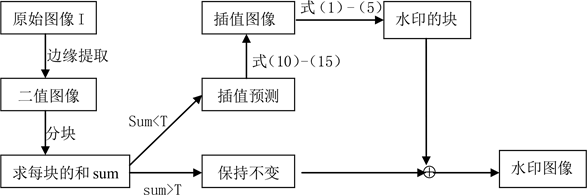Figure 2. Flowchart of embedding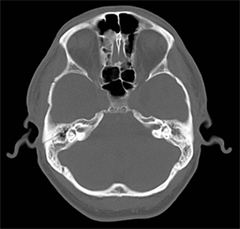(a)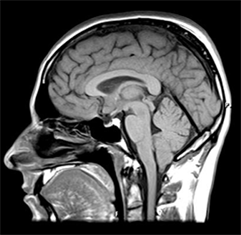(b)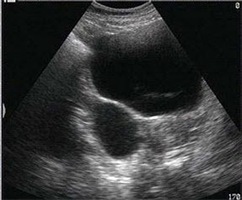(c)

Figure 3. Original image (a) CT image; (b) MRI image; (c) Ultrasound image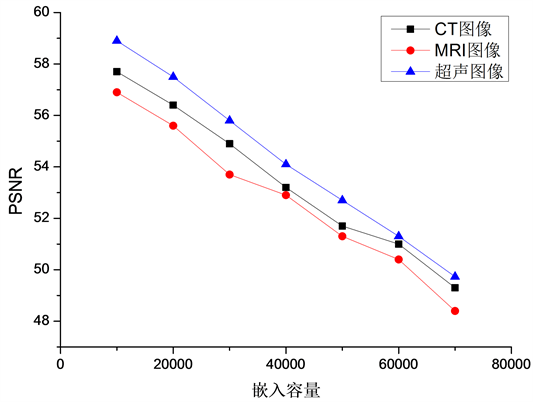Figure 4. PSNR test results for different carrier images with different embedding capacitiesTable 1. Comparison in three images of capacity and PSNR

Reversible Medical Image Watermarking Based on Image Interpolation Error Expansion[J]. 计算机科学与应用, 2018, 08(04): 480-486. https://doi.org/10.12677/CSA.2018.84053

1. 1. Hu, J.K., Chen, H.H. and Hou, T.W. (2010) A Hybrid Public Key Infrastructure Solution (HPKI) for HIPAA Privacy/Security Regula-tions. Computer Standards & Interfaces, 32, 274-280. https://doi.org/10.1016/j.csi.2009.04.005

2. 2. Tan, C.K., Ng, J.C., Xu, X.T., et al. (2011) Security Protection of DICOM Medical Images Using Dual-Layer Reversible Watermarking with Tamper Detection Capability. Journal of Digital Imaging, 24, 528-540. https://doi.org/10.1007/s10278-010-9295-4

3. 3. Deng, X.H., Chen, Z.G., Deng, X.H., et al. (2011) A Novel Dual-Layer Re-versible Watermarking for Medical Image Authentication and EPR Hiding. Advanced Science Letters, 4, 3678-3684. https://doi.org/10.1166/asl.2011.1886

4. 4. Nayak, J., Bhat, P.S., Acharya, U.R., et al. (2009) Efficient Storage and Transmission of Digital Fundus Images with Patient Information Using Reversible Watermarking Technique and Error Control Codes. Journal of Medical Systems, 33, 163-171. https://doi.org/10.1007/s10916-008-9176-2

5. 5. Vinoth, K.C. and Natarajan, V. (2016) Hybrid Local Prediction Error-Based Difference Expansion Reversible Watermarking for Medical Images. Computers & Electrical Engineering, 53, 333-345. https://doi.org/10.1016/j.compeleceng.2015.11.033

6. 6. Turuk, M.P. and Dhande, A.P. (2016) A Novel Reversible Multiple Medical Image Watermarking for Health Information System. Plenum Press, New York, NY.

7. 7. 陈亮, 刘惠文, 邓小鸿. 基于整数小波变换的数字图像可逆水印算法[J]. 计算机应用与软件, 2016, 33(4): 286-291.

8. 8. Luo, L., Chen, Z., Chen, M., et al. (2010) Reversible Image Watermarking Using Interpolation Technique. IEEE Transactions on Information Forensics & Security, 5, 187-193. https://doi.org/10.1109/TIFS.2009.2035975